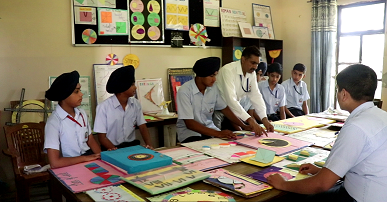Chak Alla Baksh, GT Road, Mukerian, Punjab-144211

Mathematics LabThis innovative lab enhances the student learning to explore and discover new ideas in mathematics to the fullest. The basic skills and finer nuances of mathematics can be learned in the Mathematics Laboratory. At Dasmesh Public School we believe that mathematics needs to be learned in creative ways, and not by memorization and repetition. Just as the Science lab teaches through experimentation in such a way we have our math labs, where learning is experiential for students. Our mathematical education is the method of problems solving systems through practical methods. Mathematical thinking is very difficult and we focus on the creativity necessary to make small theorems The student tries to create the solution of mathematical problems in various ways with help of math equipment.

Math Lab is a place where different concepts of Mathematics can be taught by making use of various teaching aids such as models, charts etc. Mathematical facts can be verified through activities carried out in the lab which enable the students to explore, learn and develop a favorable approach towards mathematics. The activity/experiment-based learning in Mathematics Lab helps the students to understand the subject in a simple lucid manner.

Our Math Lab is equipped with cupboards to store math tools & equipment which are issued to the students when they come to the lab for doing practical work. This approach boosts the creativity, scientific development of the brain of the children, and satisfies their zeal to do something new and unique.